• +91 9971497814
• info@interviewmaterial.com

# Chapter 5 Magnetism And Matter Interview Questions Answers

### Related Subjects

Question 1 :

Answer the followingquestions regarding earth’s magnetism:

(a) A vector needs threequantities for its specification. Name the three independent quantitiesconventionally used to specify the earth’s magnetic field.

(b) The angle of dip at alocation in southern India is about 18º.

Would you expect agreater or smaller dip angle in Britain?

(c) If you made a map ofmagnetic field lines at Melbourne in Australia, would the lines seem to go intothe ground or come out of the ground?

(d) In which direction woulda compass free to move in the vertical plane point to, if located right on thegeomagnetic north or south pole?

(e) The earth’s field, it isclaimed, roughly approximates the field due to a dipole of magnetic moment 8 ×1022 J T−1 located at its centre. Check the order of magnitudeof this number in some way.

(f ) Geologists claim thatbesides the main magnetic N-S poles, there are several local poles on theearth’s surface oriented in different directions. How is such a thing possibleat all?

(a) The three independentquantities conventionally used for specifying earth’s magnetic field are:

(i)Magnetic declination,

(ii) Angle of dip,and

(iii)Horizontal component of earth’s magnetic field

(b)The angle of dip at apoint depends on how far the point is located with respect to the North Pole orthe South Pole. The angle of dip would be greater in Britain (it is about 70°)than in southern India because the location of Britain on the globe is closerto the magnetic North Pole.

(c)It is hypotheticallyconsidered that a huge bar magnet is dipped inside earth with its north polenear the geographic South Pole and its south pole near the geographic NorthPole.

Magnetic field linesemanate from a magnetic north pole and terminate at a magnetic south pole.Hence, in a map depicting earth’s magnetic field lines, the field lines atMelbourne, Australia would seem to come out of the ground.

(d)If a compass is locatedon the geomagnetic North Pole or South Pole, then the compass will be free tomove in the horizontal plane while earth’s field is exactly vertical to themagnetic poles. In such a case, the compass can point in any direction.

(e)Magnetic moment, M =8 × 1022 J T−1

Radius of earth, r =6.4 × 106 m

Magneticfield strength,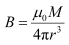Where,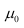= Permeability of free space =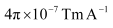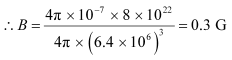This quantity is ofthe order of magnitude of the observed field on earth.

(f)Yes, there are severallocal poles on earth’s surface oriented in different directions. A magnetisedmineral deposit is an example of a local N-S pole.

Question 2 :

(a) The earth’s magneticfield varies from point to point in space.

Does it also change withtime? If so, on what time scale does it change appreciably?

(b) The earth’s coreis known to contain iron. Yet geologists do not regard this as a source of theearth’s magnetism. Why?

(c) The charged currents inthe outer conducting regions of the earth’s core are thought to be responsiblefor earth’s magnetism. What might be the ‘battery’ (i.e., the source of energy)to sustain these currents?

(d) The earth may have evenreversed the direction of its field several times during its history of 4 to 5billion years. How can geologists know about the earth’s field in such distantpast?

(e) The earth’s fielddeparts from its dipole shape substantially at large distances (greater thanabout 30,000 km). What agencies may be responsible for this distortion?

(f ) Interstellar space hasan extremely weak magnetic field of the order of 10−12 T. Can such a weak fieldbe of any significant consequence? Explain.

[Note: Exercise 5.2 is meant mainly to arouse your curiosity.Answers to some questions above are tentative or unknown. Brief answerswherever possible are given at the end. For details, you should consult a goodtext on geomagnetism.]

(a) Earth’s magnetic fieldchanges with time. It takes a few hundred years to change by an appreciableamount. The variation in earth’s magnetic field with the time cannot beneglected.

(b)Earth’s core containsmolten iron. This form of iron is not ferromagnetic. Hence, this is notconsidered as a source of earth’s magnetism.

(c)Theradioactivity inearth’s interior is the source of energy that sustains the currents in theouter conducting regions of earth’s core. These charged currents are consideredto be responsible for earth’s magnetism.

(d)Earth reversed thedirection of its field several times during its history of 4 to 5 billionyears. These magnetic fields got weakly recorded in rocks during their solidification.One can get clues about the geomagnetic history from the analysis of this rockmagnetism.

(e)Earth’s field departsfrom its dipole shape substantially at large distances (greater than about30,000 km) because of the presence of the ionosphere. In this region, earth’sfield gets modified because of the field of single ions. While in motion, theseions produce the magnetic field associated with them.

(f)An extremely weak magneticfield can bend charged particles moving in a circle. This may not be noticeablefor a large radius path. With reference to the gigantic interstellar space, thedeflection can affect the passage of charged particles.

Question 3 :

A short bar magnetplaced with its axis at 30º with a uniform external magnetic field of 0.25T experiences a torque of magnitude equal to 4.5 × 10−2 J.What is the magnitude of magnetic moment of the magnet?

Magnetic fieldstrength, B = 0.25 T

Torque on the barmagnet, T = 4.5 × 10−2 J

Angle between the barmagnet and the external magnetic field,θ = 30°

Torque is related tomagnetic moment (M) as:

T = MB sin θ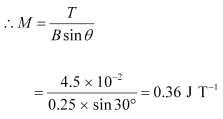Hence, the magneticmoment of the magnet is 0.36 J T−1.

Question 4 :

A short bar magnet ofmagnetic moment m = 0.32 J T−1 is placed in a uniformmagnetic field of 0.15 T. If the bar is free to rotate in the plane of thefield, which orientation would correspond to its (a) stable, and (b) unstableequilibrium? What is the potential energy of the magnet in each case?

Moment of the barmagnet, M = 0.32 J T−1

External magneticfield, B = 0.15 T

(a)The bar magnet isaligned along the magnetic field. This system is considered as being in stableequilibrium. Hence, the angle θ, between the bar magnet and themagnetic field is 0°.

Potential energy of thesystem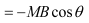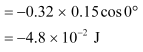(b)The bar magnet isoriented 180° to the magnetic field. Hence, it is in unstable equilibrium.

θ = 180°

Potential energy =− MB cos θ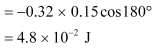Question 5 :

A closely wound solenoidof 800 turns and area of cross section 2.5 × 10−4 m2 carriesa current of 3.0 A. Explain the sense in which the solenoid acts like a barmagnet. What is its associated magnetic moment?

Number of turns in thesolenoid, = 800

Area ofcross-section, A = 2.5 × 10−4 m2

Current in thesolenoid, I = 3.0 A

A current-carryingsolenoid behaves as a bar magnet because a magnetic field develops along itsaxis, i.e., along its length.

The magnetic momentassociated with the given current-carrying solenoid is calculated as:

M = n I A

=800 × 3 × 2.5 × 10−4

= 0.6 J T−1

Question 6 :

If the solenoid inExercise 5.5 is free to turn about the vertical direction and a uniformhorizontal magnetic field of 0.25 T is applied, what is the magnitude of torqueon the solenoid when its axis makes an angle of 30° with the direction ofapplied field?

Magnetic field strength, B =0.25 T

Magnetic moment, M =0.6 T−1

The angle θ,between the axis of the solenoid and the direction of the applied field is 30°.

Therefore, the torqueacting on the solenoid is given as: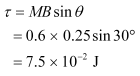Question 7 :

A bar magnet of magneticmoment 1.5 J T−1 lies aligned with the direction of a uniformmagnetic field of 0.22 T.

(a) What is the amountof work required by an external torque to turn the magnet so as to align itsmagnetic moment: (i) normal to the field direction, (ii) opposite to the fielddirection?

(b) What is the torqueon the magnet in cases (i) and (ii)?

(a)Magnetic moment, M =1.5 J T−1

Magneticfield strength, B = 0.22 T

(i)Initial angle betweenthe axis and the magnetic field, θ1 = 0°

Final angle between theaxis and the magnetic field, θ2 = 90°

The work required tomake the magnetic moment normal to the direction of magnetic field is given as: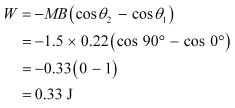(ii) Initial anglebetween the axis and the magnetic field, θ1 = 0°

Final angle between theaxis and the magnetic field, θ2 = 180°

The work required tomake the magnetic moment opposite to the direction of magnetic field is givenas: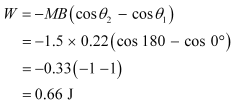(b)For case (i):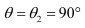Torque,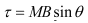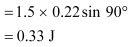For case (ii):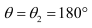Torque,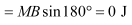Question 8 :

A closely wound solenoidof 2000 turns and area of cross-section 1.6 × 10−4 m2,carrying a current of 4.0 A, is suspended through its centre allowing it toturn in a horizontal plane.

(a) What is the magneticmoment associated with the solenoid?

(b) What is the force andtorque on the solenoid if a uniform horizontal magnetic field of 7.5 × 10−2 Tis set up at an angle of 30º with the axis of the solenoid?

Number of turnson the solenoid, n = 2000

Area of cross-section ofthe solenoid, A = 1.6 × 10−4 m2

Current in thesolenoid, I = 4 A

(a)The magnetic momentalong the axis of the solenoid is calculated as:

M = nAI

=2000 × 1.6 × 10−4 × 4

= 1.28 Am2

(b)Magnetic field, B =7.5 × 10−2 T

Angle between themagnetic field and the axis of the solenoid, θ = 30°

Torque,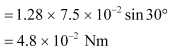Since the magnetic field is uniform, the force on the solenoid iszero. The torque on the solenoid is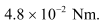Question 9 :

A circular coil of 16turns and radius 10 cm carrying a current of 0.75 A rests with its planenormal to an external field of magnitude 5.0 × 10−2 T.The coil is free to turn about an axis in its plane perpendicular to the fielddirection. When the coil is turned slightly and released, it oscillates aboutits stable equilibrium with a frequency of 2.0 s−1. What is themoment of inertia of the coil about its axis of rotation?

Number of turnsin the circular coil, N = 16

Radius of thecoil, r = 10 cm = 0.1 m

Cross-section of thecoil, A = πr2 = π ×(0.1)2 m2

Current in thecoil, I = 0.75 A

Magnetic fieldstrength, B = 5.0 × 10−2 T

Frequency ofoscillations of the coil, v = 2.0 s−1

Magnetic moment, M = NIA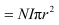= 16 × 0.75× π × (0.1)2

= 0.377 J T−1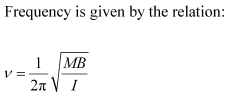Where,

I = Moment of inertia of the coil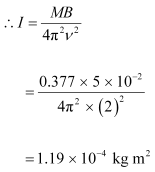Hence, the moment of inertia of the coil about its axis of rotationis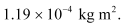Question 10 :

A magnetic needle freeto rotate in a vertical plane parallel to the magnetic meridian has itsnorth tip pointing down at 22º with the horizontal. The horizontal component ofthe earth’s magnetic field at the place is known to be 0.35 G. Determine themagnitude of the earth’s magnetic field at the place.

Horizontal component ofearth’s magnetic field, BH = 0.35 G

Angle made by the needlewith the horizontal plane = Angle of dip =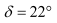Earth’s magneticfield strength = B

We can relate and BHas: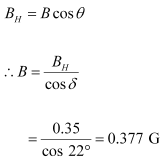Hence, the strength ofearth’s magnetic field at the given location is 0.377 G.

Todays Deals### Chapter 5 Magnetism And Matter Contributorskrishan

Name:
Email:

# Latest News# 9000 interview questions in different categories